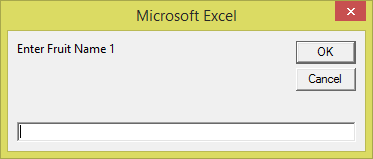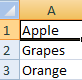### VBA MODULE 10

#### Arrays

An array is a group of variable of same data type which can store one or more values under a single name using index numbers. Each of the values in an array can be represented by an index number and these values are referred as the element of array.

Let's say you want to store a list of weekdays, you can use an array to do that. For this you can declare an array of 7 string variables to hold the names of the weekdays. If you name the array WeekDays, you can refer to the first element of the array as WeekDays(1), the second element as WeekDays(2), and so on.

## Declaring One-dimensional and Multi-dimensional Arrays

We need to declare an array before using it and this can be done using Dim statement. Unlike variables, we also need to specify the number of elements in an array.

One dimensional array uses only one index hence it can be declared as given below

one-dimentional NumberArray having 10 elements:-

 Dim NumberArray (1 to 10) as Integer

You can also choose to specify only the upper index. If you omit the lower index, VBA assumes that it’s 0. Therefore array can be defined using only upper index, see below

 Dim NumberArray(10) as Integer

If you want VBA to assume 1 rather than 0 as the lower index for your arrays, include the following statement in the Declarations section of your module:

 Option Base 1

## Example 1-

Preparing a list of employees using one-dimensional array and displaying the name of employee saved in index number 3 using msgbox() function

 Sub employeelist() Dim EmployeeList(1 To 4) As String EmployeeList (1) = "Evan" EmployeeList (2) = "Clad" EmployeeList (3) = "Wix" EmployeeList (4) = "Trod" MsgBox  EmployeeList (3) End Sub

Example 2-

Declare an array FruitName of five strings using the Dim keyword. We include an InputBox to accept input from the user. We also use the For ...Next loop to accept the input three times and display the five names from cell A1 to cell E1.

 Sub FruitName() Dim FruitName(1 To 3) As String For i = 1 To 3 FruitName(i) = InputBox("Enter Fruit Name " & i) Cells(i, 1) = FruitName(i) Next End Sub

When we run the program, an input box will appear, as shown below. This input box will repeat three times and let the user enter three names.The three names will be displayed in the spreadsheet as shown below:Multi-dimensional array uses more than one indexes. Multidimensional arrays are often needed when we are dealing with more complex database, especially those that handle large amount of data.

Declaring a multi-dimensional Rollno array where the first range is for row and second range is for column.

Dim RollNo (1 to 4, 1 to 5) as integer

Example

In this example we will use nested for next loops to enter the values in the cells using multi-dimensional array

 Sub EmployeeDesignation() Dim Employee(3, 1) As String Employee(0, 0) = "Name" Employee(0, 1) = "Designation" Employee(1, 0) = "David" Employee(1, 1) = "Manager" Employee(2, 0) = "Todd" Employee(2, 1) = "Team Leader" Employee(3, 0) = "Sam" Employee(3, 1) = "Associate" For i = 0 To 3     For j = 0 To 1         Cells(i + 1, j + 1).Value = Employee(i, j)    Next j  Next i End Sub

## Types of arrays

VBA supports two types of arrays

Static Arrays

Static arrays have a fixed number of elements which is decided during its declaration.

 Dim ArrayYear(12) As String

Dynamic Arrays

A dynamic array doesn’t have a predefined number of elements as we don’t declare the number of elements during declaration of dynamic arrays.

It is declared with empty parentheses, as follows:

 Dim DemoArray() As String

Before using this array, we have to use ReDim statement to declare that how many elements the array has. We can use the ReDim statement any number of times to change the array size as required.

 ReDim DemoArray (1 To 10)

We can use ReDim again if it is required to change the array size again in the code but to keep the values that were previously assigned to the array we need to use Preserve keyword.

The following example shows how you can preserve an array’s values when you redimension the array:

 ReDim Preserve DemoArray (1 To 10)

DemoArray currently has ten elements and if you want to execute the array using 12 elements, the first ten elements remain intact, and the array has room for two additional elements. If we reduce the size of array to 8, then first eight elements are retained but the remaining two elements will be erased.